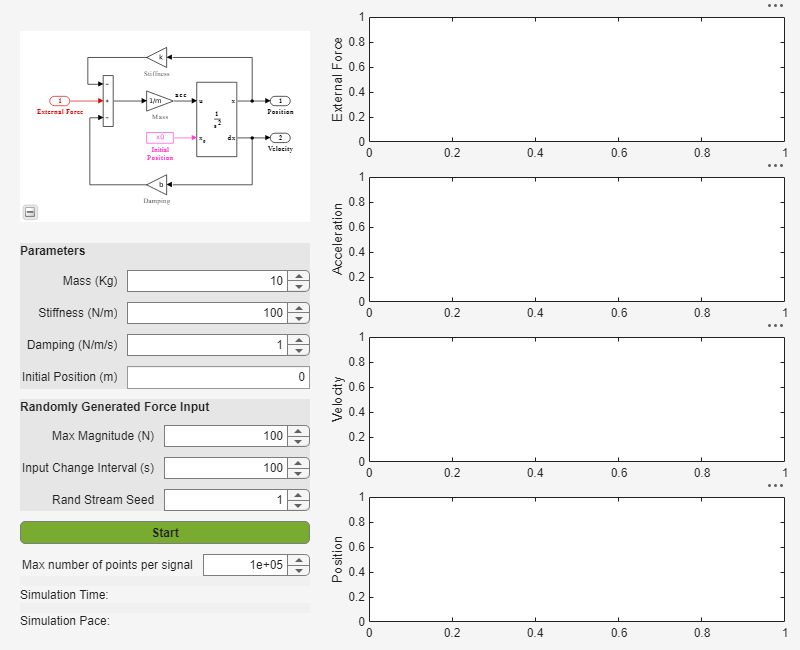# Deploying A Simulation App with Simulink Compiler

In this example, we use an app that is prepared in the App Designer and deploy it with Simulink Compiler.

### Open and Explore Model

In this example, we use the model of a mass springer damper system. The mass-spring-damper model consists of discrete mass nodes distributed throughout an object and interconnected via a network of springs and dampers. This model is well-suited for modelling object with complex material properties such as non-linearity and elasticity. In this example we use the mass spring damper system. The system is parametrized by mass (m), spring stiffness (k), damping (b) and the initial position (x0). The input to the system is the applied force.

To explore this model with different values of the tunable parameters, create the following model workspace variables:

• Mass - m.

• Spring stiffness - k.

• Damping - b.

• Initial position - xo.

To create the model workspace variables, go to the Modelling tab and select Model Workspace in the Data Repositories in the Design section. Use the Add MATLAB Variables icon to add the above four variables. Add the appropriate initial values, for example, 3, 128, 2 and 0.5 respectively.

```open_system('MassSpringDamperModel'); ```### Create the App in App Designer

Use the MATLAB APP Designer to create an app to simulate the model with different parameter values and input signals. To learn more about how to create an app using the App Designer, see Create and Run a Simple App Using App Designer Use the `MassSpringDamperApp.mlapp` file to use the app.

```MassSpringDamperApp ```App Details

The main part for the app is the simulate button callback function. It has the following salient parts: setup the `SimulationInput` object, configure it for deployment, simulate, and plot the simulation results.

The functionality of the application to change and experiment with the tunable parameters is defined in the callback function `SimulateButtonPushed`. This callback function enables you to change,experiment and analyze different simulations by modifying the values in the app designer.

### SimulateButtonPushed Callback Function Code

This section explains the code written to create the app, `MassSpringDamperApp`. The callback function `SimulateButtonPushed` is called in the app designed in the App Designer. This callback function defines how the model is simulated. We use the `Simulink.SimulationInput` object to set the variables to the model and use these variables to change the values and analyze the model.

Create the `Simulink.SimulationInput` Object in the `SimulateButtonPushed` Function

In the `SimulateButtonPushed` function, create a `SimulationInput` object, SimInp for the model `MassSpringDamperModel`. Use the `setModelParameters` method on the `SimulationInput` object. In this example, we set the `StopTime` model parameter for the simulation.Set the Values of the Tunable Parameters and the Input Signal

To set the input signal to the model, use the `ExternalInput` property of the `Simulink.SimulationInput` object, `simInp`. Use the `setVariables` method to set the values of the four tunable parameters. Create the force input signal and set it as the `ExternalInput` in the simulation input object.Configure for Deployment

Now that we have assigned all the values to the variables and set the input signal, the `Simulink.SimulationInput` object is required to be configured for deployment. Use the `simulink.compiler.configureForDeployment` function of Simulink Compiler. This function handles all the settings required for the script to be compatible for deployment by setting the simulation mode to rapid accelerator, and by setting the parameter `RapidAcceleratorUpToDateCheck` to `off`.Simulate and Plot the Results

Use the configured Simulink.SimulationInput object to run the simulation with the `sim` command. Plot the results from the simulation using the `Simulink.SimulationOutput` object, `simOut`.### Test Out the Application in App Designer

Before deploying the application, ensure that the app runs in the App Designer. Click the Simulate button on the app to verify that the application works by simulating the model for different values.

### Compile Script for Deployment

To compile the app, use the `mcc` command, followed by the script name.

`mcc -m MassSpringDamperApp.mlapp`